Open Access ArticleThis Open Access Article is licensed under a Creative Commons Attribution-Non Commercial 3.0 Unported Licence

# How can infra-red excitation both accelerate and slow charge transfer in the same molecule?†

Zheng Ma a, Zhiwei Lin d, Candace M. Lawrencef, Igor V. Rubtsov *d, Panayiotis Antoniou e, Spiros S. Skourtis *e, Peng Zhang *a and David N. Beratan*abc
aDepartment of Chemistry, Duke University, Durham, North Carolina 27708, USA
bDepartment of Physics, Duke University, Durham, North Carolina 27708, USA
cDepartment of Biochemistry, Duke University, Durham, North Carolina 27710, USA
dDepartment of Chemistry, Tulane University, New Orleans, Louisiana 70118, USA
eDepartment of Physics, University of Cyprus, Nicosia 1678, Cyprus
fDepartment of Chemistry, Xavier University of Louisiana, New Orleans, Louisiana 70125, USA

Received 8th January 2018 , Accepted 26th June 2018

First published on 27th June 2018

A UV-IR-Vis 3-pulse study of infra-red induced changes to electron transfer (ET) rates in a donor–bridge–acceptor species finds that charge-separation rates are slowed, while charge-recombination rates are accelerated as a result of IR excitation during the reaction. We explore the underpinning mechanisms for this behavior, studying IR-induced changes to the donor–acceptor coupling, to the validity of the Condon approximation, and to the reaction coordinate distribution. We find that the dominant IR-induced rate effects in the species studied arise from changes to the density of states in the Marcus curve crossing region. That is, IR perturbation changes the probability of accessing the activated complex for the ET reactions. IR excitation diminishes the population of the activated complex for forward (activationless) ET, thus slowing the rate. However, IR excitation increases the population of the activated complex for (highly activated) charge recombination ET, thus accelerating the charge recombination rate.

## I. Introduction

Infra-red (IR) excitation provides an appealing means to manipulate electron transfer (ET) reactions. Recent experimental studies have found that IR excitation can change and even redirect charge flow at the molecular scale,1–9 and theoretical studies have suggested that exciting bridge vibrations could influence electron transfer coupling pathways and their interferences.10–16 Early 3-pulse UV-IR-Vis experiments conducted on guanosine-cytidine (GC) hydrogen-bond bridged anthracene/dimethylaniline (DMA-GC-Anth) structures (Scheme 1a)1 found IR-induced ET rate slowing from DMA to (photoexcited) anthracene by about 67%. It was hypothesized that rate slowing might be caused by IR-induced weakening of the bridge mediated donor–acceptor interaction. The current study expands the IR-perturbed experiments to examine vibrational perturbations to charge recombination in DMA-GC-Anth and to establish a comprehensive theoretical understanding of the molecular origins of IR-perturbed ET rate effects.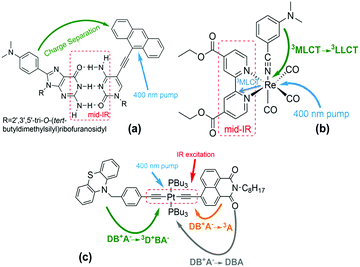Scheme 1 (a) Structure of DMA-GC-Anth1 used in the theoretical studies described here. UV excitation prepares the Anth-localized electronic excited state, followed by charge transfer from DMA to Anth. IR excitation of the hydrogen bonded bridge vibrations reduces the charge-separation rate. Other structures in previous studies (not analysed here): (b) fac-[ReI(CO)3(DCEB)(3-DMABN)] (ReEBA).9,17 UV excitation and intersystem crossing prepare a triplet electronically excited MLCT state (Re to DCEB ligand charge transfer state), and charge transfer then produces a triplet 3LLCT state (3-DMABN to DCEB charge transfer). Here, the charge transfer rate is accelerated by IR excitation of the ring-stretching bpy modes. (c) PTZ–CH2–Ph–C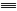C–Pt–CC–NAP.2,5 400 nm excitation and intersystem crossing prepares the electronically excited bridge-to-acceptor charge transfer state (DB+A−). IR excitation of the –CC– bridge modes decreases the forward charge transfer (DB+A− to 3D+BA−) rate and enhances the charge-recombination rate (DB+A− to 3A).

IR-perturbed charge-separation was reported recently in fac-[ReI(CO)3(DCEB)(3-DMABN)] (ReEBA) complexes (Scheme 1b), where 3DMABN is 3-dimethylaminobenzonitrile and DCEB is 4,4′-(dicarboxyethyl)-2,2-bipyridine.9,17 After formation of a triplet metal-to-ligand charge transfer (3MLCT) excited state, charge transfer proceeds to a triplet ligand-to-ligand charge transfer (3LLCT) state. The 3LLCT state has nearly full-electron transfer from 3-DMABN to DCEB.9 Excitation of the acceptor DCEB ring-stretching modes produces ET rate acceleration of ∼28%. The calculation of triplet excited electronic state energies for distortions of the normal coordinate associated with mid-IR excitation indicates that vibrational excitation of the bipyridine ring stretching drives the donor and acceptor electronic states (3MLCT and 3LLCT)17 to near degeneracy, accounting for the ET rate acceleration.9

Narrow-band IR excitation was also used to perturb ET in structures with phenothiazine (PTZ) donors, naphthalene monoimide (NAP) acceptors, and platinum(II)-trans-acetylide bridges (Scheme 1c).2,3,5 Acetylide bridge stretching modes, identified as the ET reaction coordinate, were excited.7 A vibrationally hot bridge-to-acceptor charge transfer (CT) state, DB+A, was prepared with a 400 nm UV-pump. The DB+A state has several possible fates: (1) it can form a triplet acceptor localized excited state (3A), (2) it can form a 3D+BA charge-separated state (CSS), or (3) it can undergo charge-recombination (DB+A to DBA). Excitation of the –CC– stretching motion was found to cause the forward charge-separation (DB+A to 3D+BA) to turn off, while the charge-recombination yield (DB+A to 3A) was increased. Changing the donor from PTZ–CH2 to PTZ produced weaker suppression of the forward ET rate. With OMe–PTZ as the donor, the CSS yield was reduced to <10% upon IR excitation, consistent with preliminary theoretical analysis.2,5 IR excitation of –CC– stretching was suggested to drive the DBA system past the crossing point of the DB+A and 3D+BA potential energy surface crossing for the PTZ–CH2 donor, based on TD-DFT analysis. This perspective explains the suppression of the forward ET as arising from an IR-induced decrease in the activated complex population.2,5 The surface crossing between the charge-separated and the charge transfer states is believed to become accessible when the acetylide stretch is IR-excited in the PTZ–CH2–bridge–NAP species, it is believed to be barely accessible in the IR-excited PTZ–bridge–NAP species, and is believed to be inaccessible in the IR-excited OMe–PTZ–bridge–NAP species. This trend rationalizes the distinct influences of IR excitation on charge-separation in these three systems. In PTZ–CH2–bridge–NAP and PTZ–bridge–NAP, IR excitation accelerates the back ET (from the CT to the 3A state), suggesting an IR-induced increase in the CT-to-3A electronic coupling.7 The authors suggest that IR excitation modifies the donor–acceptor energy gap and the donor–acceptor coupling, causing the ET rate to change.2,8 Recent theoretical studies of the PTZ–bridge–NAP species indicate that IR excitation increases the DA coupling and contributes to enhancing the charge-recombination rate.7

Early theoretical models of IR-perturbed ET focused on model Hamiltonians with multiple coupling pathways. Inelastic tunnelling was predicted to modulate coupling pathway interferences by leaving vibrational markers on the electron tunnelling route,10,15 enabling the switching-on of otherwise symmetry forbidden ET reactions.12,16 Recent simulations with a IR-perturbed high-frequency bridge mode (ℏωkBT) coupled to ET find that the induced donor–acceptor energy gap fluctuations may influence the ET rate significantly when: (1) D, B, and A electronic states are nearly degenerate, or (2) the ET time scale is comparable to the intramolecular vibrational redistribution (IVR) time scale.11 Other non-equilibrium rate theories based on generalizations of Fermi's golden rule explore non-equilibrium non-adiabatic dynamics.18 The comprehensive perspective developed here describes how IR excitation influences both nuclear Franck–Condon factors and the bridge-mediated electronic couplings.

IR-excitation of the H-bond vibrational modes in DMA-GC-Anth was found to slow charge-separation.1 Here, we report that IR-excitation during charge-recombination accelerates the rate. We examine four IR perturbing effects on these ET reaction rates in order to understand the origins of the opposite effects for charge separation and recombination: (1) IR-induced changes to the mean-squared DA coupling, (2) IR-induced changes to the donor–acceptor coupling fluctuations, which can change the validity of the Condon approximation, (3) IR-induced changes to the (quantum) Franck–Condon factor, and (4) IR-induced changes to the population of the activated complex. We find that IR-induced ET rate modulation in DMA-GC-Anth most likely originates from perturbations to the population of states in the Marcus curve-crossing region. The reason that IR-excitation accelerates charge-recombination in DMA-GC-Anth while slowing charge-separation arises mainly from difference in the activation energies of the two ET reactions.

## II. Measuring IR-perturbed charge recombination in DMA-GC-Anth

We performed 3-pulse UV-pump/IR-pump/Vis-probe experiments with IR pulses delayed with respect to the UV excitation. This allows the IR pulses to perturb either the charge separation or recombination. By chopping the IR pulses at half of the laser repetition rate, we monitored the difference in optical densities with and without the IR perturbation (Fig. 1). The IR pump beam was tuned to ca. 6 μm to excite carbonyl stretching and NH, NH2 bending bridge modes (Scheme 1a). The experimental details of the 3-pulse measurement are outlined in the ESI and were reported earlier.1 The measurements were performed in dichloromethane using a 130 μm path length flow cell under N2 at 23.5 ± 0.4 °C. To minimize the amount of unbound acceptor in the sample (Kassoc = 3.8 × 104 M−1),29 the measurements were performed in mixtures with a 5-fold molar excess of the donor (75 mM) over the acceptor (15 mM).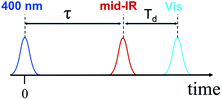Fig. 1 Pulse sequence used in the 3-pulse experiments.

The 3-pulse transient spectra for DMA-GC-Anth were measured at various time delays, Td (Fig. 2), while τ was fixed at 75.5 ps to vibrationally excite the charge separated (CS) state. They represent the spectral changes caused by vibrational excitation in the CS state. The transient spectra measured at small Td delays are very different from the transient spectra associated with the anthracene-localized excited state, but resemble the transient spectra of the CS state (Fig. S2). As in the case of the charge-separation reaction,1 the strongest transients are seen at small time delays Td (Fig. 2A). These transients are dominated by the coupling of the bridge vibrational modes to the CS state, thus representing a vibrationally hot CS state. At larger Td, the transient spectra change to represent the vibrationally relaxed CS state (Fig. 2). The shape of the transient spectrum at large delays (35.7 ps) matches well the vibrationally relaxed transient spectrum of the CS state (dark green line). The vibrational coupling contribution to the transient spectrum is expected to decay with a characteristic cooling time of the vibrationally hot CS state, which is expected to be similar to the vibronic coupling decay time of 10.6 ps observed for the anthracene-localized excited state in the acceptor-only compound.1 Indeed, the fast component of the spectral changes in Fig. 2A is ca. 10 ps (Fig. 2B). The negative sign of the transient spectra shows that less of the CS state remains in the sample at the detection time (Td) when the sample is IR excited, indicating that the rate of charge-recombination (CR) is increased when the bridge is vibrationally excited.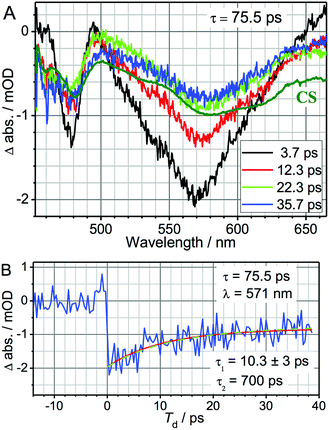Fig. 2 (A) UV-pump/mid-IR-pump/Vis-probe transient spectra of DMA-GC-Anth measured at various Td-delays (see inset) with τ = 75.5 ps to target the charge-recombination reaction. An inverted and normalized transient (UV/Vis) spectrum of the CS state (dark green) of DMA-GC-Anth is shown for comparison. (B) 3-pulse kinetics at 571 nm (blue). A fit with a two-exponential function, τ1 = 10.3 ± 3 ps and τ2 = 700 ps (fixed) is shown with a red line. The green dashed line shows the modeling results, also given in Fig. 4 (black line).

The kinetics at different wavelengths were fit to a two-exponential function with the slow decay component set to 700 ps, since it is expected to match the rate of the unperturbed charge-recombination reaction. The fast component is represented as a convolution of the vibrational cooling in the CS state (diminishing with Td) with the difference in amounts of charge-recombination products generated with and without vibrational excitation (increasing with Td). To describe the CR rate change induced by IR excitation, we use the scheme shown in Fig. 3. The superscript V labels vibrationally excited charge separated (CSV) and electronically excited (EEV) states, as well as the rates involving vibrationally excited states (kVCR, kVCS, and kV0). The rate constants, kC1 and kC2, represent vibrational cooling in the EEV and CSV states, respectively. This scheme differs from that of ref. 1 by the addition of a relaxation channel from the vibrationally excited CS state, kC2. In the modeling, kC1 and kC2 were set to 10.6 ps, which is the vibrational cooling time measured for the electron acceptor (C-Anth) sample.1 Kinetic profiles were computed for two sets of initial conditions: [EE, EEV, CS, CSV] = [0.045, 0.022, 0.50, 0.15] (solid lines) and [EE, EEV, CS, CSV] = [0.067, 0, 0.65, 0] (dashed line), which correspond to the cases with and without vibrational excitation, respectively. The populations at τ = 75.5 ps (initial conditions for the CR reaction modulation) were computed using the scheme in Fig. 3, with the quantum yield of CS, QYCS = 0.7 (see ESI),1 and vibrational excitation probabilities of 0.33 and 0.23 in the electronically excited and CS states, respectively (see ESI). The value of kVCR was varied such that the difference, ΔCS = CSV − CS, in the amount of CS states remained in the sample under the two initial conditions at the waiting time of 36 ps matched the experimentally measured amount. The latter was determined from a comparison of the amplitude of the transient spectrum in the 3-pulse measurements with the transient CS state spectrum measured under the same experimental conditions in the 2-pulse UV/Vis measurements. The modeling results in the rate of CR of kVCR ∼ (200 ± 50 ps)−1 (Fig. 4). Therefore, the IR excitation induces a ca. 3.5-fold increase of the charge-recombination rate. Note that the proposed kinetic scheme for charge recombination (Fig. 3) is very simple, involving a single recombination channel. The validity of the assumption that vibrational cooling in the CS and ES are similar could cause additional errors, but they are not expected to be large. We next examine four sources of IR-induced ET rate changes in order to understand why vibrational excitation accelerates charge-recombination but slows charge separation in DMA-GC-Anth.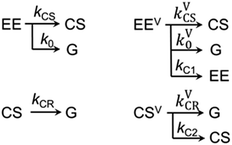Fig. 3 Kinetic scheme used to describe the three-pulse data. EE represents the acceptor localized excited electronic state and G represents the ground electronic state. The superscript “V” labels the vibrationally excited electronic states. CS is the charge separated electronic state.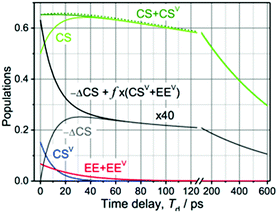Fig. 4 Modeling of the 3-pulse kinetics for τ = 75.5 ps as a function of probe time delays after the IR pulse, Td. The time evolution of the populations was computed for two initial conditions: [EE, EEV, CS, CSV] = [0.045, 0.022, 0.50, 0.15] (solid lines) and [EE, EEV, CS, CSV] = [0.067, 0, 0.65, 0] (dashed line), which correspond to the experiments with and without vibrational excitation, respectively. For the initial conditions with vibrational excitation, the CS (green) and CSV (green) populations are shown separately. The difference in the amount of CS states formed for the two initial conditions, multiplied by a factor of 40 for clarity, is shown with a grey line; the black line models the total relaxation signal at λ = 571 nm where the vibronic coupling contribution is approximated as f × (EEV + CSV) with f = −0.09 to match the data in Fig. 2B.

## III. IR-induced ET rate modulation mechanisms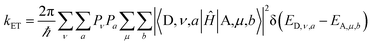(1)

Two kinds of vibrational modes are included in eqn (1): modes that modulate the donor–acceptor (DA) energy gap and modes that modulate the DA electronic couplings. Ĥ is the electronic Hamiltonian. ν and μ in eqn (1) denote quantum numbers of modes that modulate the energy gap. The indices a and b denote the quantum numbers of modes that modulate the DA electronic coupling. Pν and Pa are the populations of the two kinds of modes. ED,ν,a and EA,μ,b are the initial and final vibronic state energies, respectively. In the Condon approximation, the ET rate is: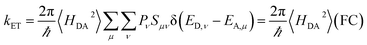(2)
where Sμν = |〈ν|μ〉|2. These equations indicate that the IR-excitation can influence the ET rate: (1) by changing the validity of the Condon approximation (Section III.2), (2) by changing the DA coupling (Section III.3), or (3) by changing the Franck–Condon factor (Sections III.4 and III.5). The physical origins of these effects are described in Fig. S3 based on a time-dependent formulation of ET theory. The time-dependent framework allows identification of characteristic time scales at play, including the ET reaction time (k−1ET), the donor–acceptor energy gap fluctuation time (τFC), the coupling fluctuation time (τc), and the IVR time (τIVR). For simplicity, each vibrational mode in this study is assumed to modulate either the DA couplings or the DA energy gap, but not both.

The Condon approximation holds when the time scale of DA coupling fluctuations, τc, is longer than the time scale of DA energy gap fluctuations (τFC, the Franck–Condon time). The Franck–Condon time is the time that the reaction coordinate persists in the configurations that produce donor–acceptor electronic energy degeneracy.13,19–28 Under thermal equilibrium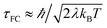(kB is the Boltzmann constant, T is the temperature, λ is the total reorganization energy. For ET with a classical reaction coordinate, λ has only classical components).13,25 Typical values of τFC are on the order of a few fs.13,25 The Condon approximation is most likely to fail in ET systems with small reorganization energies. The Condon approximation may also fail when DA coupling fluctuations are coupled to high-frequency vibrational modes, since high-frequency motion can drive DA coupling fluctuations.

The effects of IR perturbations on ET rates also depend upon the vibrational energy redistribution (IVR) time scale (τIVR) for the molecule. If vibrational excitation causes an ET rate change (by modulating the DA couplings or energy gap), the ET must be faster than the time scale for IVR to remove energy from rate perturbing modes of motion.11

### III.1. Summary of ab initio characterizations of DMA-GC-Anth

TD-DFT/B3LYP theory indicates that the second singlet electronic excited state (S2) is an anthracene-localized excitation. The H-bond equilibrium geometry for the S2 state is not significantly different from that of the ground state. The first singlet electronic excited state (S1) is a charge-separated state. The adiabatic energy difference between the S1 and S2 states (without zero-point energy corrections) is 0.33 eV, while the adiabatic energy difference between the S1 and ground states is 2.17 eV. These values are consistent with experimental ΔG values for charge separation (−0.41 eV) and recombination (−2.5 eV).29

The 3-pulse experiment on DMA-GC-Anth begins with a π → π* excitation of the anthracene fragment (S0 → S2). DFT analysis indicates that the energy gap between the DMA fragment (donor) HOMO and the bridge fragment (GC) LUMO is ∼3.5 eV in the S2 equilibrium geometry (see Table S2 and Scheme S1 in ESI), so hole-mediated superexchange is likely the charge-separation mechanism. Charge-separation occurs when an electron tunnels from the DMA π HOMO to the anthracene π HOMO via the mediating GC. Charge-recombination occurs when the electron on the anthracene π* LUMO transfers to the DMA π HOMO. At the S1 equilibrium geometry, the energy difference between the anthracene π* LUMO and the bridge-C π* orbital is ∼1 eV. Both charge-separation and recombination are mediated by virtual states of the GC bridge.

The computed inner-sphere reorganization energies (see Section II of ESI) for charge-separation (0.34 eV) and charge-recombination (0.41 eV) are similar, and the outer-sphere reorganization energies for the two reactions are expected to be similar.30 The total reorganization energy determined experimentally for charge-separation is ∼1 eV. Calculations of the DMA-GC-Anth electronic structure are described in Section II of the ESI.

### III.2. Non-Condon effects

When the DA coupling fluctuation time scale is less than the Franck–Condon time (τcτFC), non-Condon effects may arise. Troisi et al. found that non-Condon effects reduce the high-temperature ET rate when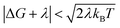(near activationless regime with classical reaction coordinate).21,23,24 Outside of this |ΔG + λ| window, non-Condon effects were found to accelerate ET. If IR-excitation accelerates coupling fluctuations, the non-Condon effects may be enhanced.

The temperature dependence of charge-separation for DMA-GC-Anth is well described using a classical reaction coordinate (see Section III.4) with λ ≈ 1 eV and ΔG = −0.41 eV.29 In this range of parameters, DMA-GC-Anth is not expected to be in the regime of non-Condon-induced rate suppression. Thus, charge-separation in DMA-GC-Anth is likely to be accelerated by IR-induced non-Condon effects. Charge-separation in DMA-GC-Anth, however, was found to be slowed by IR excitation,1 so this slowing of the ET rate is not likely to be induced by non-Condon effects.

For charge-recombination, a ΔG value of −2.5 eV was derived experimentally.29 We assume that the outer-sphere reorganization energy for charge-recombination is not significantly different from that for charge-separation, so the total reorganization energy for charge recombination is also ∼1 eV (the computed inner-sphere reorganization energies for both processes are similar). Hence, we also have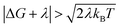for charge recombination. Therefore, non-Condon effects may contribute to accelerating charge recombination. Assuming that τc for charge recombination in the absence of IR excitation has a typical value of 25 fs,23 and that IR excitation is expected to reduce the value of τc to 5–20 fs,24 the ratio of the IR-accelerated ET rate to the unperturbed ET rate varies from 1 and 7 (see ESI Section III.1 for details). Therefore, the IR-induced ET rate acceleration caused by enhanced non-Condon effects may contribute to accelerating the charge-recombination rate in DMA-GC-Anth. Since the unperturbed charge-recombination rate is ∼(700 ps)−1, a 7-fold ET rate increase prior to IVR (assuming a ∼20 ps upper limit for the IVR time scale) would only produce a ∼17% increase in the overall charge-recombination rate. Hence, non-Condon effects do not likely explain the charge-recombination rate acceleration.

### III.3. IR-induced 〈H2DA〉 modulations

In the Condon approximation, the mean-squared DA electronic coupling enters the ET rate (eqn (2)). The ensemble averaged squared DA coupling is: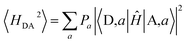(3)
where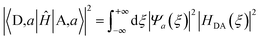(4)
and ξ is a normal coordinate along which the DA coupling varies. Ĥ is the electronic Hamiltonian. Pa is the population of vibrational state a and Ψ is the vibrational wave function. When ℏωkBT and Peqa = δa,0 (ω is the mode frequency), IR excitation creates a non-equilibrium vibrational state distribution. Consider the change of the squared DA coupling caused by geometry distortion (δξ) from the equilibrium geometry (ξeq) as a function of the distortion (i.e., fξ) = |HDA(ξeq + δξ)|2 − |HDA(ξeq)|2). If fξ) + f(−δξ) = 0, the vibrationally averaged squared coupling in eqn (3) for each mode is approximately |HDA(ξeq)|2 for any vibrational state a. In order for vibrational motion to change significantly the mean-squared DA coupling, the change of squared DA coupling caused by the distortion from equilibrium geometry should not be an odd function with respect to the geometry change.

Vibrational modes that influence 〈H2DA〉 can be populated by IVR following IR excitation. As the vibrational state population relaxes following excitation, the averaging in eqn (3) should be computed for states populated by IVR. The IVR time scale needs to be comparable to or shorter than the ET time scale for IVR to influence 〈H2DA〉 and the rate.

We varied the geometry in DMA-GC-Anth along the ground electronic state normal modes corresponding to one quantum of vibrational energy (calculations were performed between the classical turning points of the first vibrational state) and calculated the DA coupling at each geometry (see Tables S6 and S7 in ESI). The IR excited normal modes were assumed to be the same for the ground and excited electronic states. This approximation is expected to be valid since the acceptor electronic excited states are localized on the anthracene moiety, and the mid-IR excitation is largely bridge localized (see Sections I.1–I.4 in ESI).

IR excitation of DMA-GC-Anth in the three-pulse experiment was centred at 1670 cm−1, with a width of ∼120 cm−1, targeting H-bond vibrations.1 We examined 11 vibrational modes with large H-bond amplitudes that are likely to be directly excited by IR (they have large IR intensity, based on B3LYP analysis). These modes are associated largely with in-plane H-bond motion that could change the DA distance and electronic coupling. Hydrogen bond motion includes carbonyl stretching, NH2 scissoring, and in-plane bending. The percentage change in 〈H2DA〉 calculated using eqn (3) and (4) for the a = 1 state compared to the a = 0 state (using the notation of eqn (3) and (4)) was found to be <10% for any of the 11 normal modes. Since the IR photon cannot excite more than one of these 11 modes at the same time, the experimentally observed IR-induced ET rate slowing of ∼60% does not appear to arise from electronic coupling changes induced by in-plane hydrogen bond vibration of the GC bridge.

We also examined coupling changes induced by 18 out-of-plane H-bond modes (600–800 cm−1) that may be excited by IVR (these modes were selected based on the magnitudes of their out-of-plane motion). These modes could plausibly change the donor–acceptor orientation and coupling. The computed charge-separation couplings for the a = 0 and a = 1 vibrational states differ by less than 10%, indicating that out-of-plane H-bond vibrational excitation does not likely explain the observed IR-induced charge-separation rate reduction (the injected vibrational energy is not enough to excite more than three of these out-of-plane H-bond modes simultaneously). The donor–acceptor coupling in DMA-GC-Anth is largely pi-mediated. IR excitation largely perturbs the σ-symmetry H-bonds (e.g., through in-plane scissoring modes) in the GC bridge, which do not couple substantially to the π-symmetry pathways and result in little change in the donor–acceptor distance and orientation. We also analysed normal modes in the 1300–1500 cm−1 frequency range, as they are likely to be excited by IVR. No significant changes were found in the couplings computed when these modes were excited (see Tables S2 and S3 in ESI).

The percentage changes in 〈H2DA〉 upon vibrational excitation of each of the modes mentioned above were also computed for the charge-recombination reaction. We found coupling changes are less than 10% upon exciting bridging H-bond vibrations. Therefore, the rate perturbation for both charge-separation and charge-recombination in DMA-GC-Anth are likely not rooted in the changes of ensemble averaged squared DA couplings.

### III.4. IR-induced changes to the quantum Franck–Condon factor

We now explore how non-thermal state populations influence Franck–Condon factors. In the mixed quantum-classical regime with one quantum mode, the ET rate in the Condon approximation is: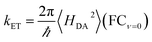(5a)
and the Franck–Condon factor is31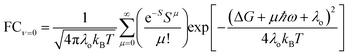(5b)
λo is the outer-sphere reorganization energy (the reorganization energy contributed by classical modes), ΔG is the ET reaction free energy, and S is the Huang–Rhys factor for the quantum mode with vibrational frequency ω (S = λi/ℏω, where λi is the inner-sphere reorganization energy contributed by the quantum mode, and the total reorganization energy is λ = λi + λo).31–33 IR excitation of the quantum mode coupled to its first vibrational excited state (ν = 1) changes the Franck–Condon sum in eqn (5b) to: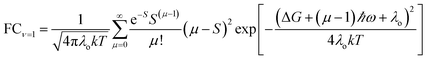(6)

For ET from the ground vibrational state (eqn (5b)), the largest term in the Franck–Condon sum of eqn (5b) occurs for μS. Eqn (6), in contrast, has a dip as a function of the final state quantum number μ. Differences between the Franck–Condon factors of eqn (5) and (6) are illustrated in Fig. 5 (for a vibrational mode of 1200 cm−1, outer-sphere reorganization energy λo = 0.7 eV and Huang–Rhys factor S = 1 or 2) as a function of ΔG. Near ΔG + λo + λiℏω ≈ 0, IR-excitation slows the ET rate. Outside of this ΔG window, IR-excitation enhances the ET rate.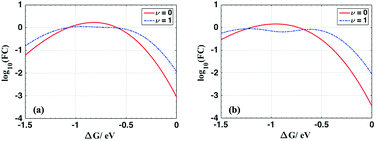Fig. 5 Plot of log10(FCν=0) (red solid lines) and log10(FCν=1) (blue dotted lines) as a functions of ΔG for λo = 0.7 eV and (a) S = 1; (b) S = 2 at room temperature with ℏω = 1200 cm−1. The ET rate is predicted to decrease in the near activationless regime (ΔG + λi + λo − ℏω ≈ 0) due to IR excitation of the quantum reaction coordinate and to increase elsewhere.

The size of the rate change produced by excitation to the ν = 1 state depends on the ET parameters. In general, IR induces ET rate suppression with ΔG + λo + λiℏω ≈ 0 and rate acceleration otherwise (see Fig. S4 of the ESI and associated discussions). The magnitude of the IR-induced rate suppression can be as large as ∼10-fold for ℏω = 500–1700 cm−1 and S ≤ 2.5 (see Table S8 in ESI). FCν=0 is dominated by the maximum value in the eSSμ/μ! sum, while FCν=1 is dominated by the maximum value in the eSS(μ−1)(μS)2/μ! sum. As a result, high-frequency quantum modes with the large S values produce substantial rate slowing with IR excitation because the maxima in the eSS(μ−1)(μS)2/μ! sum is smaller than the maximum in eSSμ/μ! as S grows (see Fig. S8 in ESI).

For each combination of ℏω, S and λo, there is a ΔG range where IR excitation induces rate suppression (ΔG + λo + λiℏω ≈ 0). The width of this ΔG range is ∼0.2 to 0.7 eV for ℏω = 500–1700 cm−1, λo = 0.2–0.6 eV, and S ≤ 2.5 (see Table S8 in ESI). This rate suppression window widens as S grows. In the temperature range where eqn (5) and (6) are valid, rate suppression is larger at lower temperatures.

When ΔG is positive and large, or λi is large (compared to ℏω), such that ΔG + λi + λoℏω, IR excitation accelerates the ET rate. In this regime, for each given μ value, the exponential factor (exp[−(ΔG + (μ − 1)ℏω + λo)2/4λokBT], eqn (6)) in FCν=1 is always larger than (exp[−(ΔG + μℏω + λo)2/4λokBT], eqn (5b)), and the rate from the IR populated ν = 1 state is larger than the rate from the ν = 0 state. This IR-induced rate acceleration is similar to the effect produced by simple heating. When −ΔG is very large or λi is small (ΔG + λi + λoℏω, λi > kBT), the exponential terms in the summations of eqn (5b) and (6) peak when μ is large. In this case (ΔG + λi + λoℏω), eSS(μ−1)(μS)2/μ! in eqn (6) is always larger than eSSμ/μ! in eqn (5b). Therefore, FCν=1 is larger than FCν=0, and IR excitation increases the ET rate when ΔG + λi + λoℏω. In summary, when ΔG + λi + λoℏω, for a mixed classical-quantum ET mechanism, IR-excitation slows the ET rate. When ΔG + λi + λoℏω or ΔG + λi + λoℏω, IR-excitation accelerates the ET rate.

Charge-separation in DMA-GC-Anth. The measured temperature-dependent charge-separation rates29 were fitted to eqn (5), and the parameters were input to eqn (6) to compute the ET rate with IR excitation to the first vibrational excited state (kν=1ET). We explored vibrational frequencies of the quantum mode from 250 cm−1 to 1600 cm−1, and ΔG from −0.1 eV to −1.0 eV. The outer sphere reorganization energy was varied from 0.2 to 0.8 eV. Parameters for eqn (6) obtained from nonlinear fitting are not unique, and the fitted values of λi range from 0.2 eV to several electron volts. None of the fitted ET parameter combinations produce IR-perturbed charge-separation rates that are smaller than the unperturbed rate. Therefore, nonlinear fitting does not predict IR induced ET rate suppression, consistent with our understanding of the ET parameters regime where IR-induced rate suppression would be expected, based on eqn (5b) and (6). Thus, the Franck–Condon factor changes induced by vibrational excitation do not explain the experimentally observed charge-separation rate slowing in these compounds. Indeed, the temperature dependence of the observed charge-separation rate is better fit by a classical Marcus-like high-temperature Franck–Condon expression (eqn (7)) rather than by the mixed quantum-classical rate equation (eqn (5)). These findings indicate that charge-separation in DMA-GC-Anth is likely governed by IR-perturbation of the classical reaction coordinate, which we explore in the next section.
Charge-recombination in DMA-GC-Anth structure. The reorganization energies for charge-recombination and charge-separation are approximately the same, based on analysis of experimental ET kinetic data1,29 and our calculations (see ESI Section II). Our DFT analysis finds that the inner-sphere reorganization energy is dominated by the DMA-G fragment for both charge-separation and charge-recombination. The inner-sphere reorganization energy λi has contributions from the DMA-G fragment (λi,D) and from the Anth-C fragment (λi,A). For the charge-separation reaction, we found λi,D = 0.3 eV and λi,A = 0.06 eV. For charge-recombination, we found λi,D = 0.26 eV, λi,A = 0.12 eV.

The decomposition of the inner-sphere reorganization energy by vibrational modes indicates that the contributions from each mode for charge-recombination and charge-separation are similar (see Table S8 in ESI). Thus, charge-recombination is also likely to be controlled by classical reaction coordinate motion. Hence, the IR induced change in the quantum Franck–Condon factor is not likely to modulate the charge-recombination rate.

### III.5. IR-induced changes to the classical (Marcus) nuclear factor

The high-temperature non-adiabatic ET rate is: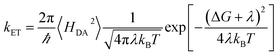(7)
The ET rate increases as the time in the crossing region,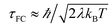, grows.30,34,35 This term appears as a prefactor in the Marcus ET rate expression.13,25 IR excitation and subsequent IVR produce non-equilibrium populations of the classical reaction coordinate and faster DA energy gap fluctuations (reducing τFC). Excitation of the classical reaction coordinate motion changes the DA energy gap probability distribution function (ρUDA)) near the surface crossing. The solid black lines in Fig. 6 indicate the probability density for the DA energy gap at thermal equilibrium (ρeqUDA)), and the dotted lines represent a possible non-equilibrium probability density of the DA energy gap (ρneqUDA)) induced by IVR. For near activationless ET (Fig. 6, left), the surface crossing occurs near the bottom of the donor potential energy surface. Energy flow into classical reaction coordinate motion by IVR increases the nuclear coordinate velocity near the minimum of the donor surface, reduces the time that the donor and acceptor spend in energy degeneracy, and decreases the ET rate. For a highly activated ET reaction (Fig. 6, right), IVR excitation of classical reaction coordinate motion increases the probability of reaching the curve crossing region and grows the ET rate.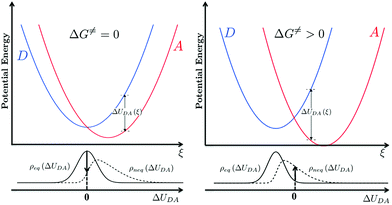Fig. 6 IVR induced change to the probability density of energy gaps near the DA surface crossing associated with a classical reaction coordinate. ΔUDA(ξ) is the donor–acceptor energy gap as a function of reaction coordinate ξ. ρeq(ΔUDA) is the density of state as a function of ΔUDA at thermal equilibrium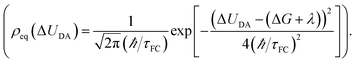IVR excitation of classical reaction coordinates produces a non-equilibrium density of states (ρneq(ΔUDA)). For an activationless reaction (left, ΔG = 0), a more rapidly decaying energy gap correlation function induced by IVR would deplete population from the curve crossing region and lead to a decrease in the ET rate. For an activated ET reaction (right, ΔG > 0), more rapid fluctuations of the energy gap may increase the population near the curve crossing and effectively enhance the ET rate.

The non-adiabatic ET rate in the high-temperature regime can be written (see details in ESI Section III.4 and ref. 36):

 kET = 〈transition velocity〉 × 〈density of states at curve crossing per unit length〉 (8)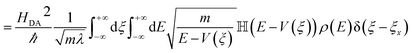(9)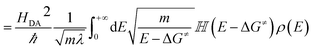(10)
ξ is the reaction coordinate, and the donor and acceptor potential energy surfaces cross at ξ = ξx. V(ξ) is the donor potential energy surface and V(ξx) = ΔG. λ is the total reorganization energy, m is the reduced mass, E is the total energy of the reaction coordinate, ρ(E) is the energy probability distribution function, and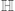is the Heaviside step function. In thermal equilibrium, ρ(E) is the Boltzmann distribution function and eqn (10) reduces to the Marcus classical rate. IVR introduces energy to the reaction coordinate and creates a non-equilibrium reaction coordinate distribution function. Because of IVR, the density of state (DOS) is increased at higher energies and the DOS is decreased at lower energies. Therefore, for near activationless ET (ΔG ≈ 0), IVR causes the value of the integral in eqn (10) to decrease (because of depletion of the DOS near the curve crossing), producing ET rate slowing. This is because the inverse of the nuclear velocity,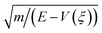(eqn (9)), decreases as E grows. That is, the IVR-induced non-equilibrium distribution for the reaction coordinate increases the nuclear velocity near the curve crossing and reduces the ET rate. For highly activated ET, ρ(E ≈ ΔG) ≈ 0 prior to IVR and kET ≈ 0. If the vibrational energy introduced to the reaction coordinate by IVR increases the value of ρ(E ≈ ΔG), the ET rate will accelerate.

The difference between the equilibrium and non-equilibrium ET rates may also be described in the language of effective activation free energies (see ESI Section III.4 for detail).37–39 For example, a general formulation of transition state rate theory was used to show that, far from equilibrium, the non-equilibrium rate can be described with a product of an exponential activation factor and a pre-factor.37 The exponential factor is associated with an effective reaction barrier.37 The height of this effective barrier depends on the non-equilibrium distribution function ρneq(E,t). Hence, IVR-induced ET rate modulation may be interpreted as a result of changed activation free energy, i.e. for IR-perturbed ET, kneqET ∝ exp[−(ΔG + δG)/kBT] and δG is the change of activation free energy caused by the non-equilibrium distribution. For highly activated ET, δG is negative, while near activationless ET reactions have positive δG values. The specific value of δG depends on the form of ρneq(E,t), but δG cannot exceed the vibrational energy introduced to the system by IR excitation.

Charge transfer in DMA-GC-Anth. The activation free energy for charge-separation in DMA-GC-Anth is approximately 0.09 eV, based on fits using a high-temperature (Marcus) Franck–Condon factor.1 The small reaction activation free energy indicates that IR excitation and subsequent IVR produce a non-equilibrium population distribution for the classical reaction coordinate that likely depletes the density of states near the curve crossing. This rationalizes a decrease in rate induced by IR excitation. The change in the IR-perturbed initial density of reaction coordinate states near the curve crossing can be estimated (quantitatively) from the DA energy gap correlation function that is computed from non-equilibrium classical molecular dynamics simulations. The charge-recombination in DMA-GC-Anth has ΔG ≈ −2.5 eV,1,29 and a reorganization energy of ∼1.0 eV, so the reaction is inverted with an activation free energy of ∼0.6 eV. The large reaction activation free energy indicates that the IVR following IR excitation injects energy into the classical reaction coordinate and vibrational states closer to the crossing point will be populated, increasing the charge-recombination rate. This IR effect is analogous to transient heating of the reaction coordinate.

### III.6. Perspectives on IR-perturbed ET kinetics

We have analysed four potential mechanisms of ET rate suppression in DMA-GC-Anth (Sections III.2–III.5). Here, we summarize the analysis in the context of a time-dependent perspective for the reactions (summarized in Fig. S3 of the ESI). This framework provides insights for designing systems with IR-perturbed ET rates. Two classes of mechanisms are included in this discussion: IR-induced modulation of the DA couplings and IR-induced modulation of the DA energy gap. For DA energy gap modulation, IR excitation and subsequent IVR produce non-equilibrium population distributions of the reaction coordinate and thus change the ET rate. For DA couplings in the Condon regime, IR-excitation and subsequent IVR can influence the mean-squared DA couplings and thus modulate the ET rate. IR and subsequent IVR can also accelerate DA coupling fluctuations and invalidate the Condon approximation.
Modulating the DA energy gap in the high-temperature (classical Marcus) regime. Assuming that IR-excitation and subsequent IVR does not drive highly anharmonic nuclear motion, IR perturbation is expected to accelerate highly activated ET reactions and to slow nearly activationless reactions. This behaviour arises from promoting/depleting reaction coordinate population in the donor–acceptor curve crossing region. This effect is similar to the effect produced by sudden heating where the reaction coordinate is far from equilibrium with its environment. In this circumstance, the Marcus ET rate expression does not apply and it is necessary to use a non-equilibrium ET rate expression (eqn (8)–(11)). Analysing non-equilibrium ET rates, we found that reactions with activation free energy on the order of kBT (near activationless) are likely to be slowed by IR-excitations. The magnitude of slowing is likely on the order of several-fold, depending upon the energy provided to the reaction coordinate. For highly-activated ET, a small reduction in the effective value of ΔGG) can produce a significant increase of the ET rate in the time period when the vibrational energy injected by IR-excitation persists in the reaction coordinate. However, the overall ET-rate acceleration may be reduced by the (small) ratio of the short IVR time scale (the dwell time for the IVR injected energy in the relevant reaction coordinate) compared to the longer ET time scale. For example, in the case of DMA-GC-Anth, a δG value of ∼0.1 eV can cause a ∼25 folds increase in the charge-recombination rate within the IVR time scale, leading to a ∼3.5-fold increase in the overall ET rate.
Mixed quantum-classical ET. If the quantum mode(s) also contribute to the reaction coordinate, the ET rate is described by a semi-classical rate expression (eqn (5)). IR-excitation of quantum mode(s) that contribute to the reaction coordinate creates a non-equilibrium reaction coordinate population distribution that can influence the ET rate. This kind of IR-induced ET rate modulation effect can accelerate or slow ET, depending on the values of ΔG, λo, λi and ℏω. We found that IR excitation of the quantum reaction coordinate produces ET rate suppression when ΔG + λi + λoℏω ≈ 0 and produces rate acceleration otherwise. With typical S values ≤ 2.5,40 and a quantum mode with vibrational frequencies between 500 and 1700 cm−1, the IR-induced rate suppression can be as large as a factor of ∼10, and the width of the ΔG window for IR-induced rates suppression is ∼0.2 to 0.7 eV. This rate suppression window widens as S grows.

The influence of vibrational excitation on ET reactions with quantum reaction coordinates was studied by Spears,41 where all inner-sphere molecular modes were treated quantum mechanically. Spears found that vibrational excitation of the quantized reaction coordinate motion causes the ET rate to be slowed for near activationless ET and accelerated for activated ET. This is consistent with the results of our analysis, where we assume that only one quantum mode contributes to the reaction coordinate. Ivanov et al. also examined the influence of vibrational relaxation on ET rate constants and transition probabilities.39,42 In the latter analysis, individual state-to-state vibronic transitions were studied.

IR-modulated DA couplings. IR-excitation can modulate the ET rate by changing the DA coupling distribution. This effect is significant when the change of donor–acceptor squared coupling caused by geometry distortion is not an odd function with respect to the distortion along the normal modes that modulate the coupling. Molecules with varying DA couplings of this kind include p-phenylenevinylene oligomers,43,44 where the DA couplings depend on the torsion angles of the donor, bridge and acceptor. Dimethylaniline-pyrene structures45 linked in a C-clamp fashion also have torsion angle dependent DA couplings, where the torsion angle between donor and acceptor dictates whether or not solvent molecules mediate the DA interaction.
IR-enhanced DA coupling fluctuations. When the Condon approximation fails (i.e., the DA coupling fluctuation time scale is shorter than or comparable to the DA energy gap fluctuation time scale), IR-excitation and subsequent IVR can accelerate the DA coupling fluctuations (enhancing non-Condon effects) and can change the ET rate. Non-Condon effects can accelerate or slow ET, depending on the ET parameters. For near activationless ET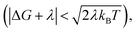fast fluctuations of the DA coupling slow the ET rate, while highly activated ET reactions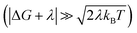are likely to be accelerated by the IR-enhanced non-Condon effects (see discussions in Section III.2 and ESI Section III.1). Non-Condon effects are most likely to arise in floppy structures where the DA coupling is strongly geometry dependent. For example, a recent computational study showed significant non-Condon effects in Fe(II)/Fe(III) self-exchange where the DA couplings were modulated by orientational changes of the ions that are induced by high-frequency vibrational excitations.46 Theoretical and experimental studies of DNA-mediated ET found that DA couplings could be modulated by vibrational excitation.47–51 Changes to both the donor–acceptor energetics and to the nucleobase orientations contribute to the DA coupling fluctuations.

## IV. Conclusions

We have examined IR-perturbed charge separation and recombination in DMA-GC-Anth. We find that IR-excitation of H-bond bridging modes accelerates the charge-recombination rate (∼3.5 fold) and slows charge separation. We pinpointed the origins of the observed IR-induced rate changes as being perturbations to the density of states of the reaction coordinate at the curve crossing. The forward ET reaction is near activationless, so IR excitation and subsequent IVR accelerate the DA energy gap fluctuations and reduce the density of states at the curve crossing, leading to rate suppression (that is, IR excitation diminishes the population of the activated complex). The charge-recombination reaction in the species studies is highly activated. IR excitation of the classical reaction coordinate motion increases the probability of the donor and acceptor energies to remain in degeneracy, resulting in IR-induced ET rate enhancement (that is, IR excitation increases the population of the activated complex). We also found that IR excitation induces faster DA coupling fluctuations (non-Condon effects) and may contribute to the charge-recombination rate acceleration, but such contributions from non-Condon effects are not expected to be substantial.

The IR-induced ET rate modulation found in DMA-GC-Anth is different from the IR-induced charge-separation rate suppression found in PTZ–bridge–NAP molecules (DB+A to 3D+BA, Scheme 1c).2,3,5 In the PTZ–bridge–NAP structures, quantum vibrational modes contribute substantially to the ET reaction coordinate while the DBA-GC-Anth reaction coordinate is largely classical. Photo-induced charge transfer in DMA-GC-Anth does not involve triplet electronic excited states and does not have multiple competing ET pathways, which makes the ET kinetics simpler to interpret in the case of DMA-GC-Anth. The fact that IR-excitation produces opposite modulation effects for charge-separation and charge-recombination in DMA-GC-Anth makes the system particularly informative.

Our analysis of the potential mechanisms for IR-induced ET rate modulations in DMA-GC-Anth provides principles for designing structures with IR-modulated ET kinetics. Near activationless ET reactions are most likely to be slowed by IR-excitation, while highly-activated ET is most likely to be accelerated (with or without quantum modes contributing to the reaction coordinate). In the Condon regime, mean-squared coupling modulations induced by IR-excitation (or subsequent IVR) may also contribute to the ET rate changes. The change in the squared DA coupling caused by the IR-induced geometry change should not be an odd function with respect to the geometric distortion in order for the mean-squared DA couplings to be modulated by IR or IVR perturbations. IR or IVR-induced acceleration of the DA coupling fluctuations may cause the Condon approximation to fail, thus changing the ET rate. While near activationless ET is likely to be slowed by non-Condon effects while highly activated ET is likely to be accelerated, non-Condon effects have been found to be weak in most ET structures. Focusing on ET structures where the ET time scale is comparable to the IVR time scale is probably the most productive strategy for discovering strong IR-induced ET rate effects. For structures that undergo slow ET, IR or IVR-induced ET rate modulations need to be significant within the IVR window so that substantial IR-induced perturbations are manifested in the overall ET rate.

## Conflicts of interest

There are no conflicts to declare.

## Acknowledgements

The authors thank the National Science Foundation awards CHE-1565812 (DNB) and CHE-1565427 (IVR) for support of this research. The authors also thank the Cyprus Research Promotion Foundation for financial support of the project via the research Grant “Vibrational Control of Electron Transfer ΔIEΘNHΣΣTOXOΣ-0311/04.” S. S. S. thanks the People Programme (Marie Curie Actions) of the European Union’s Seventh Framework Programme (FP7/2007–2013), under the Research Executive Agency Grant 609305. S. S. S. and D. N. B. also thank the Institute of Advanced Studies at the University of Freiburg, Germany for support.

## References

1. Z. W. Lin, C. M. Lawrence, D. Q. Xiao, V. V. Kireev, S. S. Skourtis, J. L. Sessler, D. N. Beratan and I. V. Rubtsov, J. Am. Chem. Soc., 2009, 131, 18060–18062 CrossRef PubMed.
2. M. Delor, T. Keane, P. A. Scattergood, I. V. Sazanovich, G. M. Greetham, M. Towrie, A. J. H. M. Meijer and J. A. Weinstein, Nat. Chem., 2015, 7, 689–695 CrossRef PubMed.
3. M. Delor, I. V. Sazanovich, M. Towrie and J. A. Weinstein, Acc. Chem. Res., 2015, 48, 1131–1139 CrossRef PubMed.
4. M. Delor, P. A. Scattergood, I. V. Sazanovich, T. Keane, G. M. Greetham, A. J. H. M. Meijer, M. Towrie, A. W. Parker and J. A. Weinstein, Physical Chemistry of Interfaces and Nanomaterials XIV, Proc. SPIE, 2015, 9549 Search PubMed.
5. M. Delor, P. A. Scattergood, I. V. Sazanovich, A. W. Parker, G. M. Greetham, A. J. H. M. Meijer, M. Towrie and J. A. Weinstein, Science, 2014, 346, 1492–1495 CrossRef PubMed.
6. P. A. Scattergood, M. Delor, I. V. Sazanovich, M. Towrie and J. A. Weinstein, Faraday Discuss., 2015, 185, 69–86 RSC.
7. X. Yang, T. Keane, M. Delor, A. J. Meijer, J. Weinstein and E. R. Bittner, Nat. Commun., 2017, 8, 14554 CrossRef PubMed.
8. I. V. Rubtsov, Nat. Chem., 2015, 7, 683–684 CrossRef PubMed.
9. Y. K. Yue, T. Grusenmeyer, Z. Ma, P. Zhang, R. H. Schmehl, D. N. Beratan and I. V. Rubtsov, Dalton Trans., 2015, 44, 8609–8616 RSC.
10. S. S. Skourtis, D. H. Waldeck and D. N. Beratan, J. Phys. Chem. B, 2004, 108, 15511–15518 CrossRef.
11. P. Antoniou, Z. Ma, P. Zhang, D. N. Beratan and S. S. Skourtis, Phys. Chem. Chem. Phys., 2015, 17, 30854–30866 RSC.
12. H. Carias, D. N. Beratan and S. S. Skourtis, J. Phys. Chem. B, 2011, 115, 5510–5518 CrossRef PubMed.
13. S. S. Skourtis, I. A. Balabin, T. Kawatsu and D. N. Beratan, Proc. Natl. Acad. Sci. U. S. A., 2005, 102, 3552–3557 CrossRef PubMed.
14. S. S. Skourtis and D. N. Beratan, Adv. Chem. Phys., 1999, 106, 377–452 Search PubMed.
15. S. S. Skourtis and D. N. Beratan, AIP Conf. Proc., 2007, 2, 809–812 CrossRef.
16. D. Q. Xiao, S. S. Skourtis, I. V. Rubtsov and D. N. Beratan, Nano Lett., 2009, 9, 1818–1823 CrossRef PubMed.
17. Y. Yue, T. Grusenmeyer, Z. Ma, P. Zhang, R. H. Schmehl, D. N. Beratan and I. V. Rubtsov, J. Phys. Chem. A, 2014, 118, 10407–10415 CrossRef PubMed.
18. R. D. Coalson, D. G. Evans and A. Nitzan, J. Chem. Phys., 1994, 101, 436–448 CrossRef.
19. D. N. Beratan and J. J. Hopfield, J. Chem. Phys., 1984, 81, 5753–5759 CrossRef.
20. J. N. Onuchic, D. N. Beratan and J. J. Hopfield, J. Phys. Chem., 1986, 90, 3707–3721 CrossRef.
21. J. Tang, J. Chem. Phys., 1993, 98, 6263–6266 CrossRef.
22. E. S. Medvedev and A. A. Stuchebrukhov, J. Chem. Phys., 1997, 107, 3821–3831 CrossRef.
23. A. Troisi, A. Nitzan and M. A. Ratner, J. Chem. Phys., 2003, 119, 5782–5788 CrossRef.
24. H. Nishioka, A. Kimura, T. Yamato, T. Kawatsu and T. Kakitani, J. Phys. Chem. B, 2005, 109, 15621–15635 CrossRef PubMed.
25. S. S. Skourtis, D. H. Waldeck and D. N. Beratan, Annu. Rev. Phys. Chem., 2010, 61, 461–485 CrossRef PubMed.
26. S. S. Skourtis, J. Lin and D. N. Beratan, in Modern Methods for Theoretical Physical Chemistry of Biopolymers, ed. J. P. Lewis and S. Tanaka, Elsevier Science, Amsterdam, 2006, pp. 357–382 Search PubMed.
27. W. R. Cook, R. D. Coalson and D. G. Evans, J. Phys. Chem. B, 2009, 113, 11437–11447 CrossRef PubMed.
28. W. R. Cook, D. G. Evans and R. D. Coalson, Chem. Phys. Lett., 2006, 420, 362–366 CrossRef.
29. J. L. Sessler, M. Sathiosatham, C. T. Brown, T. A. Rhodes and G. Wiederrecht, J. Am. Chem. Soc., 2001, 123, 3655–3660 CrossRef PubMed.
30. R. A. Marcus and N. Sutin, Biochim. Biophys. Acta, 1985, 811, 265–322 CrossRef.
31. J. Ulstrup and J. Jortner, J. Chem. Phys., 1975, 63, 4358–4368 CrossRef.
32. N. R. Kestner, J. Logan and J. Jortner, J. Phys. Chem., 1974, 78, 2148–2166 CrossRef.
33. M. Bixon and J. Jortner, Adv. Chem. Phys., 1999, 106, 35–202 Search PubMed.
34. M. D. Newton, Chem. Rev., 1991, 91, 767–792 CrossRef.
35. A. Nitzan, Chemical Dynamics in Condensed Phases, Oxford University Press, Oxford, UK, 2006 Search PubMed.
36. H. Sumi, in Electron Transfer in Chemistry, Wiley-VCH Verlag GmbH, 2008, pp. 64–108 Search PubMed.
37. H. D. Feng, K. Zhang and J. Wang, Chem. Sci., 2014, 5, 3761–3769 RSC.
38. M. H. Cho and R. J. Silbey, J. Chem. Phys., 1995, 103, 595–606 CrossRef.
39. S. V. Feskov, V. A. Mikhailova and A. I. Ivanov, J. Photochem. Photobiol., C, 2016, 29, 48–72 CrossRef.
40. J. Pieper and A. Freiberg, in The Biophysics of Photosynthesis, ed. J. Golbeck and A. van der Est, Springer New York, New York, NY, 2014, pp. 45–77 Search PubMed.
41. K. G. Spears, J. Phys. Chem., 1995, 99, 2469–2476 CrossRef.
42. V. A. Mikhailova and A. I. Ivanov, J. Phys. Chem. C, 2017, 121, 20629–20639 CrossRef.
43. W. B. Davis, M. A. Ratner and M. R. Wasielewski, J. Am. Chem. Soc., 2001, 123, 7877–7886 CrossRef PubMed.
44. W. B. Davis, W. A. Svec, M. A. Ratner and M. R. Wasielewski, Nature, 1998, 396, 60–63 CrossRef.
45. J. M. Nadeau, M. Liu, D. H. Waldeck and M. B. Zimmt, J. Am. Chem. Soc., 2003, 125, 15964–15973 CrossRef PubMed.
46. M. G. Mavros, D. Hait and T. Van Voorhis, J. Chem. Phys., 2016, 145, 214105 CrossRef PubMed.
47. F. C. Grozema, S. Tonzani, Y. A. Berlin, G. C. Schatz, L. D. A. Siebbeles and M. A. Ratner, J. Am. Chem. Soc., 2008, 130, 5157–5166 CrossRef PubMed.
48. E. Hatcher, A. Balaeff, S. Keinan, R. Venkatramani and D. N. Beratan, J. Am. Chem. Soc., 2008, 130, 11752–11761 CrossRef PubMed.
49. S. H. Lin, M. Fujitsuka and T. Majima, Angew. Chem., Int. Ed., 2016, 55, 8715–8717 CrossRef PubMed.
50. S. H. Lin, M. Fujitsuka and T. Majima, J. Phys. Chem. B, 2016, 120, 660–666 CrossRef PubMed.
51. A. A. Voityuk, K. Siriwong and N. Rosch, Phys. Chem. Chem. Phys., 2001, 3, 5421–5425 RSC.

### Footnote

Electronic supplementary information (ESI) available: Additional experimental and computational details and data. Additional details of IR-perturbed ET rate theory. See DOI: 10.1039/c8sc00092a
 This journal is © The Royal Society of Chemistry 2018